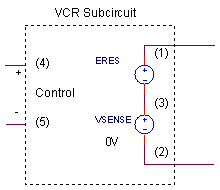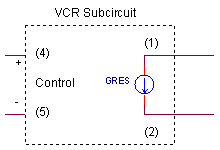eCircuit  Center

### Voltage-Controlled Resistor

CIRCUITA common request from SPICE users is a voltage-controlled resistor. Although there's no SPICE component that directly models this device, you can easily put one together. There's several ways to do it. You can even extend the device to model more complex resistance functions like RTDs and thermistors.

A RESISTOR-LESS RESISTOR MODEL

An important step in modeling a voltage-controlled resistor is creating a model of a resistor without using a resistor. But how? Think of the resistor's behavior when current is passed through it. A voltage is produced according to Ohm's Law V = I x R. Place the resistor in the circuit - it does the job automatically. But, let's rip out the resistor and do the job manually using other SPICE components. In its place, simply sense the current I and generate the voltage V = I x R with a voltage source. Here's a resistor-free subcircuit that models a 10k resistor..SUBCKT RES_10K 1 2
ERES   1  3  VALUE = { I(VSENSE)*10K }
VSENSE 3  2  DC 0
.ENDS

To sense current, SPICE uses a voltage source VSENSE (0V). It's set to 0V so there's no effect on the output voltage. The other source, ERES, generates the voltage across the "resistor" based on the sensed current times your desired resistance VALUE={I(VSENSE)*10K}.

A VOLTAGE-CONTROLLED RESISTOR

A simple addition to the above resistor model gives you a voltage-controlled resistor. All you need is a controlling voltage to multiply the resistor value: V = I x R x Vc. Here's a subcircuit for this device..SUBCKT VC_RES1_10K 1 2  4 5
ERES   1  3  VALUE = { I(VSENSE)*10K*V(4,5) }
VSENSE 3  2  DC 0V
.ENDS

As you can see, the voltage applied to nodes 4 and 5 scales the resistance value.

CIRCUIT INSIGHT   Simulate the SPICE circuit VC_RESISTOR1.CIR. Device XVCR1 calls subcircuit VC_RES_10K, a 10k Ω voltage-controlled resistor. Control voltage VCONT sweeps from 0.1  to 1.0 V. As a result, we expect the resistance to change from 1k to 10 kΩ.

To measure the XCVR1's resistance, we inject a 1A current from IS. The resulting voltage is really a measure of the device's resistance V = I x R = 1 x R = R. Plot the voltage (or measured resistance) at V(1). Does the resistance vary from 1k to 10k as expected?

VCR - VERSION 2

This time let's use a current source, GRES, to create the voltage-controlled resistor. Again our aim is to defend and uphold Ohm's  Law. But because GRES is a current output device, we rearrange the law as I = V / (R x Vc). The schematic and subcircuit look like this..SUBCKT VC_RES2_10K 1 2 4 5
GRES  1  2  VALUE = { V(1,2) / (10K*V(4,5)) }
.ENDS

GRES senses the voltage and outputs a current through the same two terminals, 1 and 2.

CIRCUIT INSIGHT   Run a simulation of VC_RESISTOR1.CIR. This time place a * in front of XVCR1 to remove it from the circuit. Remove the * before XVCR2 and RD2 to place them in the circuit. XVCR2 the calls subcircuit VC_RES2_10K. Does this model produce the 1k to 10 kΩ resistance change as version 1? If you wish, place XVCR1 in the circuit with XVCR2. Since both model the same resistance, the parallel combination should be one half of 10k.

What's up with the component RD2? Because IS and GRES are current sources, there's no DC conductance to ground. SPICE does not like this condition - the simulation will halt. To keep the algorithm happy, but avoid effecting our measured resistance, we place a large "dummy" resistor from node 1 to ground.

HANDS-ON DESIGN   Choose a different value of resistance instead of 10k. Pick a different controlling voltage range in the VCONT statement. (Example: Sweep VCONT from 1 to 5V and watch the resistance change from 10k to 50 kΩ.)

VCR - VERSION 3

Before the VALUE function came as standard equipment in the SPICE engine, designers needed to assemble a voltage-controlled resistor from the basic nuts and bolts of SPICE. The folks at Microsim (the original maker's of PSpice) published a circuit similar to the one below..SUBCKT VC_RES3_10K  1 2  4 5
ERES   1  3  POLY(2) (6,0) (4,5) 0 0 0 0 1
VSENSE 3  2  DC 0V
*
FCOPY  0  6  VSENSE 1
RRES   6  0  10K
.ENDS

It looks much the same as our first model. However, the VALUE statement has been replaced by the POLY function, available since the early days of SPICE. Again, the goal is to realize
V = I x R x Vc with simple components instead of an equation. As before, VSENSE, senses the current through our device. But this time, current source FCOPY makes a duplicate of the sensed current and pushes it through resistor RRES to produce V = I x R. Finally, ERES multiplies the voltage across RRES, V(6), by the controlling voltage V(4,5) accomplishing our goal of
V = I x R x Vc.

CIRCUIT INSIGHT   Place a * in front of XVCR1 and XVCR2. Remove the * before XVCR3 and run a simulation. Does it achieve the 1k to 10 kΩ resistance change as the previous models?

HANDS-ON DESIGN   Pick a new value for the resistance RRES. Modify the SPICE file and take your new voltage-controlled resistor out for a test drive.

SIMULATION NOTES

Each one of the models above has its advantages. Because the version 1 is a voltage source, it doesn't need a dummy resistor to ground, like version 2, when driven by a current source. On the other hand, you couldn't connect version 1 directly across a voltage source. This would create a voltage loop forcing SPICE to stop and reprimand you for breaking the rules. To correct this, you could add a small resistor in series or use VCR version 2. Obviously version 3 is handy if your SPICE version is not equipped with the VALUE function.

I'm sure there's more ways to create voltage-controlled resistor. If you come across any, please drop us a line, we're curious to see it.

Review SPICE Subcircuits at the SPICE Basics page.

See the voltage-controlled resistor (VCR) in action. The sensor in the Sensor Bridge is modeled by a VCR.

The Thermistor Model is easily developed by extending the VCR model.

SPICE FILE

Download the file or copy this netlist into a text file with the *.cir extention.

```VC_RESISTOR1.CIR - VOLTAGE-CONTROLLED RESISTOR
*
* CONTROL VOLTAGE
VCONT	10	0	PWL(0MS 0.1V	 100MS 1.0V)
RD10	10	0	1MEG
*
* CURRENT SOURCE
IS	0	1	DC	1
*
* VC RESISTOR
XVC1	1 0	10 0	VC_RES1_10K
*
*XVCR2	1 0	10 0	VC_RES2_10K
*RD2	1	0	100MEG
*
*XVC3	1 0	10 0	VC_RES3_10K
*
*
*** VC RESISTOR SUBCIRCUIT ********************
*	RESISTOR - 1,2     CONTROL - 4,5
*
.SUBCKT VC_RES1_10K	1 2	4 5
ERES	1	3	VALUE = { I(VSENSE)*10K*V(4,5) }
VSENSE	3	2	DC	0V
.ENDS
*
*
*** VC RESISTOR USING A CURRENT SOURCE *********
*	RESISTOR - 1,2     CONTROL - 4,5
*
.SUBCKT VC_RES2_10K	1 2	4 5
GRES	1	2	VALUE = { V(1,2) / (10K*V(4,5)) }
.ENDS
*
*
*** VC RESISTOR USING BASIC SPICE COMPONENTS ***
*	RESISTOR - 1,2     CONTROL - 4,5
*
.SUBCKT VC_RES3_10K   1  2   4  5
EOUT	1	3	POLY(2) (6,0) (4,5) 0 0 0 0 1
VSENSE	3	2	DC	0V
*
FCOPY	0	6	VSENSE 1
RRES	6	0	10K
.ENDS
*
* ANALYSIS
.TRAN 	0.1MS  100MS
*
* VIEW RESULTS
.PRINT	TRAN 	V(1)
.PROBE
.END```